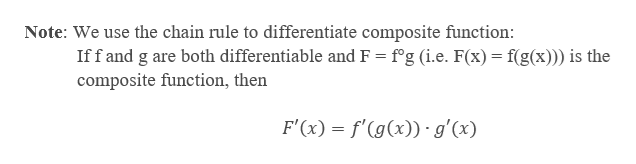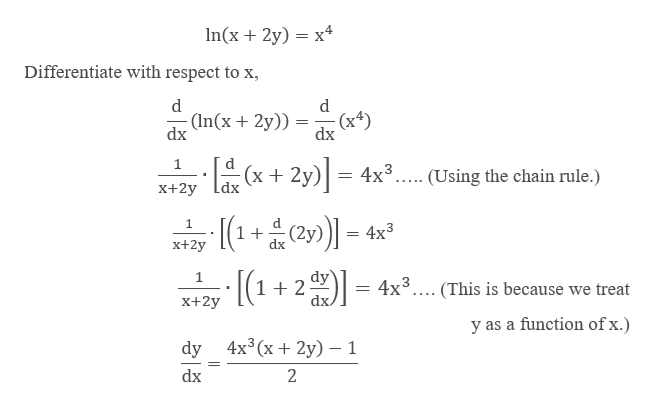What is ln(x+2y)=x^4 using implict differentiation to find the derivative of y with respect to x?

Question

What is ln(x+2y)=x^4 using implict differentiation to find the derivative of y with respect to x?

Step 1

Given function:

Step 2

In implicit differentiation, we differentiate each side of an equation by treating one of the variables as a function of the other. We treat y as an implicit function of x.help_outlineImage TranscriptioncloseNote: We use the chain rule to differentiate composite function: Iff and g are both differentiable and F fg (i.e. F(x) f(g(x))) is the composite function, then F'(x) f'(g(x))g'(x fullscreen
Step 3

Now, we differentiate th...help_outlineImage Transcriptionclosen(x 2y) x4 Differentiate with respect to x d d - (In(x2y)) (x4) dx dx 1 (x 2y) dx 4x3 (Using the chain rule.) X+2y 1 4x3 (2y) 1 + x+2y [(1+2) dy 1 4x3 . (This is because we treat dx. X+2y y as a function of x.) 4x3 (х + 2y) — 1 dy dx 2 fullscreen

Want to see the full answer?

See Solution

Want to see this answer and more?

Our solutions are written by experts, many with advanced degrees, and available 24/7

See Solution
Tagged in

Derivative